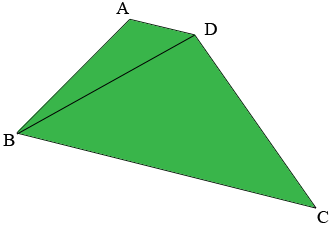SEARCH HOMEMath Central Quandaries & QueriesQuestion from Kerri: Can you please show the formula used to calculate areas as provided on this page: Calculating the area (acreage) of a four-sided lotHi Kerri,

You have a four-sided lot (quadrilateral) and you know the lengths of the four sided and one other piece of information, either the length of a diagonal or the measure of one of the angles. The calculation proceeds in two cases.

Case 1: You know the length of a diagonal.

Suppose you know the length of the diagonal $BD$ in the diagram below.You have the quadrilateral partitioned into two triangles and for each triangle you know the lengths of its three sides. In this situation you can use Heron's Formula to calculate the areas of each of the triangles and then the area of the quadrilateral is the sum of the areas of the triangles.

Case 2: You know the measure of an angle.

Suppose you know the measure of the angle $BCD$ in the diagram above. In this situation you can use the Law of Cosines to calculate the length of the diagonal $BD$ and then proceed as in case 1.

I hope this helps,
PennyMath Central is supported by the University of Regina and The Pacific Institute for the Mathematical Sciences.